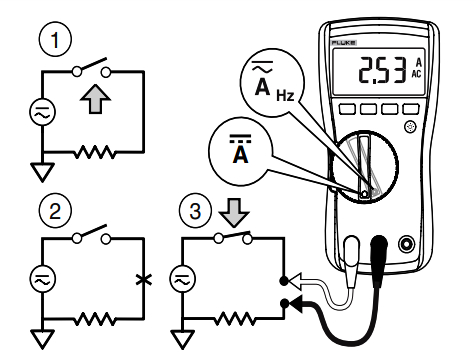Technical Article

# In-Line Ammeter Applications and Measurement Procedures

November 05, 2021 by Alex Roderick

## Learn how to best utilize an in-line ammeter and what applications they are used in.

In-line ammeters provide the most accurate current measurements when measuring currents less than 1A. In-line ammeters are the most accurate because the meter is connected in series with the load, and every milliamp of current that flows through the load must flow through the meter.

Some applications require that the in-line method of current measurement be used because in-line is the only way or best way to test a circuit or component. The in-line method of current measurement is typically used only for applications requiring very accurate current measurements and having less than 1 A of current.

Note:

In-line current measurements are the most dangerous measurement to take of any electrical measurement (voltage, resistance, clamp-on current measurements). Any in-line current measurement requires that extreme caution be used.

## In-Line Ammeter Applications

Forklift electrical circuits draw large amounts of current because forklift circuits operate at low voltages (the lower the voltage, the higher the required current for any given power). For example, a 100 W safety strobe light designed for a 36 V system requires 2.77 A to deliver 100 W of power.

$I=\frac{P}{E}$

Where

$$I = current (in amps)$$

$$P = power (in watts)$$

$$E = voltage (in volts)$$

$I=\frac{100}{36}=2.77A$

However, when a forklift or automobile is turned OFF, and all accessories (hydraulic valves, lights, radio) are OFF, the battery should have very little current flow (a little current can be drawn by digital clocks, computers, and security systems). The small amount of current that is drawn should not drain a battery that is in good shape for several weeks. However, when a load is faulty (as when a hydraulic valve solenoid stays energized, a glove box lamp remains on, or water is in the safety light or tail-lamp holder) or insulation is damaged, the battery can be drained when the forklift or automobile is OFF in a couple of hours. To test the drain (current draw) on a battery, an in-line ammeter is used. See Figure 1.##### Figure 1. To test the drain on a battery, an in-line ammeter is used.

Only fused in-line ammeters set to the highest current measuring range can be used.

To test the current draw on a battery, the ignition switch is turned to the OFF position, and all accessories are turned OFF, the positive battery post is disconnected from the battery, and an in-line ammeter is connected between the battery post and the disconnected terminal.

In-line ammeters accurately measure the small amount of current drawn from batteries. To isolate a problem or test each circuit, the fuses of the forklift or automobile can be removed one at a time.

## In-Line Ammeter Measurement Procedures

Care is required to protect the in-line ammeter, circuit, and electrician when measuring AC or DC current with an in-line ammeter. Standard safety precautions must be followed when using an in-line ammeter. The following are the standard procedures for taking an AC current measurement with an in-line ammeter:

1. Test the ammeter fuses following manufacturer-recommended procedures.

2. Verify that the expected circuit current measurement is less than the current rating (setting) of the in-line ammeter. Start with the highest current-measuring range of the in-line ammeter when the circuit current is unknown. When the circuit current can exceed the rating of the in-line ammeter, use a clamp-on ammeter, or do not take the current measurement.

3. Set the function switch of the in-line ammeter to the proper setting for measuring AC current and set the function or selector switch to the proper current rating because most ammeters include more than one current setting (A, mA, µA).

4. Verify that the test leads of the in-line ammeter are connected to the proper jacks for measuring the correct amount of AC current. Most ammeters include more than one current jack.

5. Verify that the power to the circuit being tested is OFF before connecting and disconnecting the in-line ammeter test leads. When necessary, take voltage measurements to ensure that the circuit is de-energized.

6. Do not change the function switch position on an in-line ammeter while the meter is connected to an energized circuit.

7. Turn power to the test circuit and in-line ammeter OFF before changing any ammeter settings.

8. Connect the in-line ammeter in series with the load(s) to be measured. Never connect an ammeter in parallel (as with a voltmeter) with the load(s) to be measured.

9. Turn the power ON to the circuit being tested.

10. Read the AC current measurement displayed.

11. Turn the power OFF to the circuit and in-line ammeter and remove the in-line ammeter from the circuit.

Warning

Always ensure that the function switch position matches the jack connections of the test leads. In-line ammeters are damaged when the test leads are connected to measure current and the function switch is set for a different measurement such as voltage or resistance. Some meters have an input warning function that provides a constant audible warning (beep) when the test leads are connected in the current jacks, and a noncurrent mode is selected by the function switch.##### Figure 2. AC or DC current can be measured with an in-line ammeter using standard procedures. Image courtesy of Fluke

Several test instruments include a fuse in their current-measuring circuits to protect against damage caused by excessive current. Before using an in-line ammeter, verify that the meter is fused on the current range being used. Most in-line ammeters are marked as fused or not fused at the test lead current terminals. When an in-line ammeter is not marked as fused, consider the meter unusable for measuring current. Use a clamp-on ammeter or use a clamp-on current probe accessory instead of an unfused meter. DC current is measured with an in-line ammeter using similar standard procedures (see Figure 2).